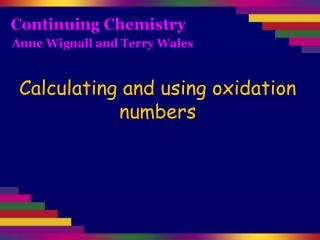DownloadDownload PresentationCalculating and using oxidation numbers

# Calculating and using oxidation numbers

Télécharger la présentation## Calculating and using oxidation numbers

- - - - - - - - - - - - - - - - - - - - - - - - - - - E N D - - - - - - - - - - - - - - - - - - - - - - - - - - -
##### Presentation Transcript

1. Calculating and using oxidation numbers

2. 1 The oxidation number of any free, uncombined element is zero. This includes polyatomic molecules of elements such as H2, O3 and S8. 2 The charge on a simple (monatomic) ion is the oxidation number of the element in that ion. In a polyatomic ion, the sum of the oxidation numbers of the atoms in that ion is equal to the charge on the ion. 3 In compounds (whether ionic or covalent), the sum of the oxidation numbers of all atoms in the compound is zero. 4 The oxidation number of oxygen is –2, except in the case of peroxides where it is –1. 5 The oxidation number of hydrogen is +1, except in the case of metallic hydrides where it is –1.

3. State the oxidation numbers for each element in this equation: Cl2(g) + 2Br-(aq) → 2Cl-(aq) + Br2(aq) 0 -1 -1 0 1 The oxidation number of any free, uncombined element is zero. 2 The charge on a simple (monatomic) ion is the oxidation number of the element in that ion. Each Br- ion has an oxidation number of -1: Br- doesn’t have an ON of -2 because there are two of them.

4. State the oxidation numbers for each element in this equation: -2 -2 2MnO4- + 3H2O2 + 2H+→ 2MnO2 + 3O2 + 4H2O Hydrogen peroxide 1 The oxidation number of any free, uncombined element is 0. 5 The oxidation number of hydrogen is +1, 4 The oxidation number of oxygen is –2 except… in the case of peroxides where it is –1. 2 In a polyatomic ion, the sum of the oxidation numbers of the atoms in that ion is equal to the charge on the ion. MnO4-: Mn + 4(-2) = -1 Mn - 8 = -1 Mn = +7 3 In compounds (whether ionic or covalent), the sum of the oxidation numbers of all atoms in the compound is zero. MnO2: Mn + 2(-2) = 0 Mn - 4 = 0 Mn = +4 0 +1 +1 +1 -2 -2 -2 -1 +7 +4

5. Oxidation number goes down from +7 to +4: MnO4- has been reduced. +7 -2 +1 -1 +1 +4 -2 0 +1 -2 2MnO4- + 3H2O2 + 2H+→ 2MnO2 + 3O2 + 4H2O Oxidation number goes up from -1 to 0: H2O2 has been oxidised.